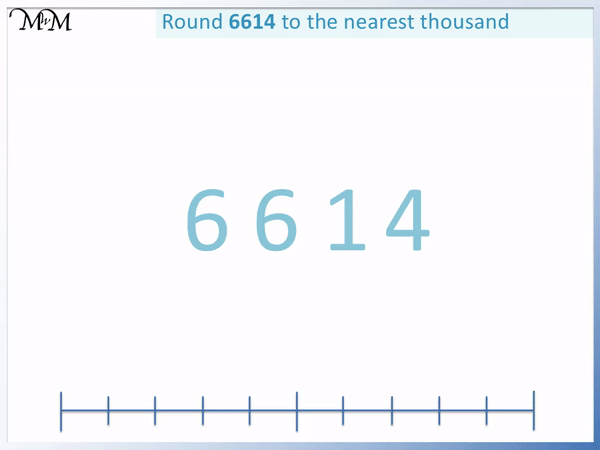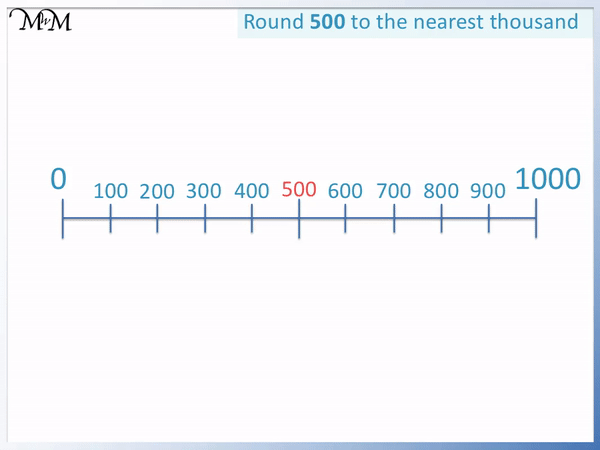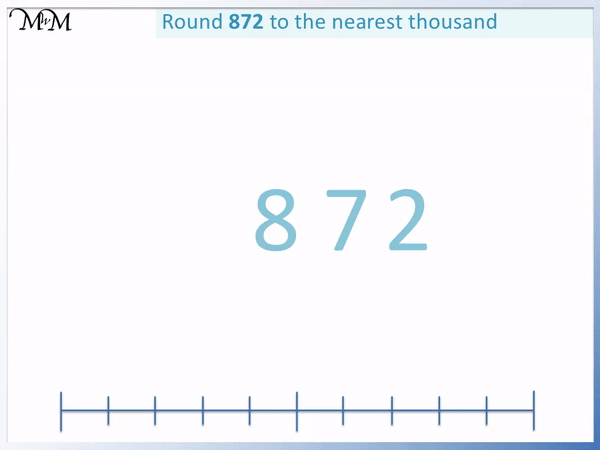# Rounding to the Nearest Thousand

Rounding to the Nearest Thousand#### Rounding to the Nearest Thousand Summary• Rounding to the nearest thousand means to write down the multiple of 1000 that is nearest to our number.
• The thousands digit will either remain the same or it will increase by one.
• 6614 is in between 6000 and 7000, so the options are to round down to 6000 or round up to 7000.
• To decide whether we round up or down, we look at the digit in the hundreds column.
• If the digit is 4 or less, we round down.
• If the digit is 5 or more, we round up.
• This is because 6500 is halfway between 6000 and 7000.
• 6614 has a 6 as its hundreds digit and 6 is ‘5 or more’.
• This means that 6614 rounds up to 7000.
• This means that 6614 is nearer to 7000 than 6000.
• We always look at the hundreds digit to decide how to round our number to the nearest hundred.

Look at the digit in the hundreds column.

If it is 4 or less, round down. If it is 5 or more, round up.• 4392 has a 4 as its thousands digit so we can choose to round down to 4000 or round up to 5000.
• We look at the digit in the hundreds column, which is 3.
• This digit is ‘4 or less’ and so we round down.
• 4392 rounds down to 4000.
• This means that 4392 is nearer to 4000 than it is to 5000.
• We can see that it is less than 4500, which is the halfway point between 4000 and 5000.# Rounding Numbers to the Nearest Thousand

## What does Rounding to the Nearest Thousand Mean?

Rounding a number to the nearest thousand means to write down the closest multiple of 1000 to the number. If the nearest multiple of 1000 is larger than the number, we say that we round up. If the nearest multiple of 1000 is less than the number, we say that we round down.

Below is the number 300.

It is in between 0 and 1000 but which is nearer?300 is nearer to zero than it is to 1000.

This is because it is less than 500, which is halfway between 0 and 1000.

We say that 300 rounds down to zero.

Here is 600.

Again, we will see if it rounds down to 0 or up to 1000.We can see that 500 is directly in between zero and 100.

We must decide whether it rounds up to 1000 or rounds down to 0.Even though 500 is directly between 0 and 1000, we say we round it up.

This is because numbers like 501, 502, 503 etc are all nearer to 1000 than to 0.

We can make a rule for rounding that all numbers with a 5 or more in the hundreds column round upwards to the next thousand.

Because 501, 502, 503 etc are all nearer to 1000 than to 0, they all round up to 1000.

It makes sense to round 500 up as well.We say that all numbers with a ‘5 or more’ in the hundreds column round up to the next thousand.

All numbers with a ‘4 or less’ in the hundreds column round down to the previous thousand.

Basically, in each thousand, if we have any number up to 499, it rounds down. If we have any number from 500 to 999, then we round up.

When teaching rounding to the nearest thousand, it is helpful to use a number line to show the relative size of each number. We can use it to see which thousand the number is nearest to.

However, once this concept is understood, it is better to look at the digits and use our rounding rule to decide whether to round up or down.

Here is 872. We need to decide whether to round down to 0 or up to 100.

We look at the hundreds digit to decide.

We have 8 hundreds in 872.8 is ‘5 or more’, so we round up.

872 rounds up to 1000 because it is nearer to 1000 than it is to 0.

## How to Round to the Nearest Thousand

To round to the nearest thousand:

2. If this digit is 4 or less, then round down.
3. If this digit is 5 or more, then round up.

To round up, increase the digit in the thousands column by one and set the hundreds, tens and ones digits to zero.

To round down, keep the digit in the thousands column the same and set the hundreds, tens and ones digits to zero.

For example we will round 4392 to the nearest thousand.

The first step is to look at the digit in the hundreds column.

4382 has a 3 in the hundreds column.

3 is ‘4 or less’ and so we round 4392 down.To round 4392 down, we keep the thousands digit the same and set the hundreds, tens and ones digits to zero.

We keep the 4 as 4 and set the 3,9 and 2 to zero.

4392 rounds down to 4000.

This means that 4392 is nearer to 4000 than it is to 5000.

In this example, we have 6614.

We first read the digit in the hundreds column.

We have a 6 in the hundreds column, which is ‘5 or more’.The rule for rounding tells use that if the digit in the hundreds column is ‘5 or more’ then we round up to the next thousand.

We increase the 6 to a 7 and set the following digits to zero.

6614 rounds up to 7000, which means that it is nearer to 7000 than it is to 6000.

In this example of rounding to the nearest thousand we have 29803.

We can see that we have 29 thousands in 29803.

This means that we have the choice of rounding between 29 thousand and 30 thousand.

We either round 29803 down to 29000 or round it up to 30000.We use our rounding rule to decide how to round this number. We look at the digit in the hundreds column of 29803.

We have an 8 in the hundreds column of 29803.

8 is ‘5 or more’ and so, we round up.

We round up to 30 thousands, which is written as 30000.

29803 is nearer to 30000 than it is to 29000.Now try our lesson on Roman Numerals 1 to 10 where we learn how read Roman numerals from 1 to 10.